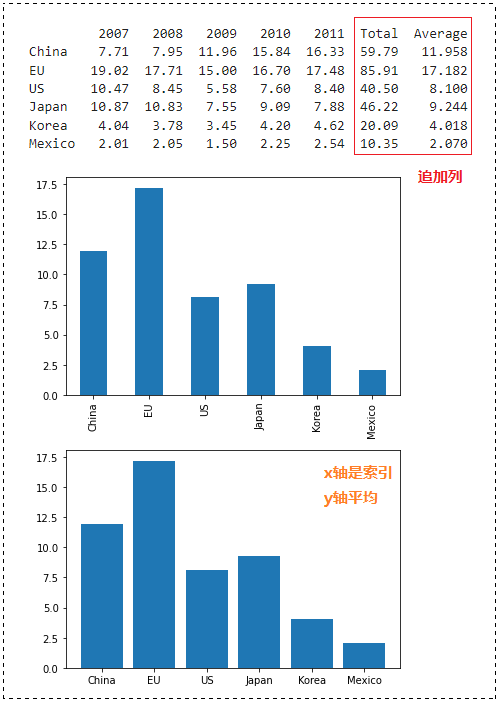### pandas 介绍

Pandas 是什么？熊猫？14年网龄的我，早就在互联网发表情包了，这种熊猫表情包我可太熟悉了。

” pandas 就是一个用于数据处理和分析的 python 库 ”

pandas 支持读取和处理各种外部资源数据，比如读取 CSV 文件、文本文件、Excel 文件、web 数据等，还可以使用 matplotlib 可视化数据。先提一嘴 pandas 的数据结构

• 一维数据结构：Series
• 二维数据结构：Data Frame
• 三维数据结构：Panel

pandas 在数据中支持多种运算函数，使用 pandas 库需要引入头文件：

`import pandas as pd`一般会 as pd 一下，这样用起来能更方便些。（就像 numpy 我们习惯去 as np 一样）

numpy 数组是所有元素都相同的数据类型，但 pandas 允许元素的数据类型不同，并生成结构数，比如 Series 和 DataFrame

### Series 数据结构简单的一维数据结构，能展示出带有索引 (index) 的一维数组。

import pandas as pd
data = [1, 3, 5, 7, 9]
# 不指定
s1 = pd.Series(data)
# 指定index
s2 = pd.Series(data, index = [“安娜”, “末日铁拳”, “麦克雷”, “莱因哈特”, “禅雅塔”])
print(s1, “\n\n”, s2, “\n”)
print(s2.values) # 只打印值
print(s2.index) # 只打印索引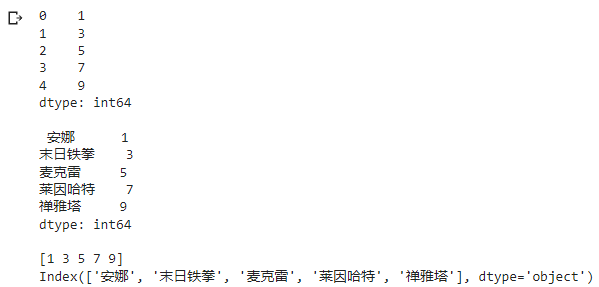import pandas as pd
# 利用字典构造 series
data = pd.Series (
{“苹果”: “Apple”, “橘子”: “Orange”, “香蕉”: “banana”, “桃子”: “peach”}
)
print(data)
print(data.index) # 打印索引
print(data.values) # 打印值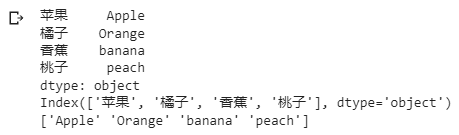### DataFrame 数据结构二维的表格型数据结构，DataFrame 接受带行和列的表数据。

• index 和列具有各自的标签（名称）
• 每个列可以有不同的数据类型
• 默认情况下，按列管理数据

• 字典、列表、nd-array 对象、Series 数据结构
• 使用 pd.DataFrame() 函数创建
• 生成的默认索引为从 0 开始的整数
• 可使用 index 关键字命名索引
• 可使用 columns 关键字命名列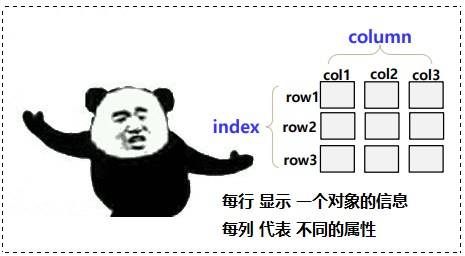import pandas as pd
data = [
[2019, 2.8, “1.63M”],
[2020, 3.4, “1.75M”],
[2021, 5.5, “1.84M”]
]
# 什么都不指定
df1 = pd.DataFrame(data)
print(df1, “\n”)
# 指定 index 和 columns
df2 = pd.DataFrame (
data, # 数据
index = [1, 2, 3], # 指定索引
columns = [‘year’, ‘GDP_rate’, ‘GDP’] # 指定列
)
print(df2)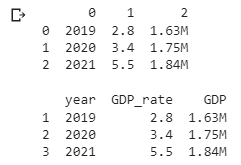import pandas as pd
data = {“year” : [2019, 2020, 2021],
“GDP_rate” : [2.8, 3.4, 3.0],
“GDP” : [“1.63M”, “1.75M”, “1.83M”]
}
## data 是一个字典
df = pd.DataFrame(data) # 等同于 df = pd.DataFrame(data=data)
print(df, “\n”)
print(df.iloc, “\n”) # row 引用类型：df.loc[index名称]，df.iloc[index编号]
print(df[“year”], “\n”) # 等同于 print(df.year)
# column 引用方法：df[“列名”] 或 df.列名
## 查看数据类型
print(type(df.iloc))
print(type(df[“year”]))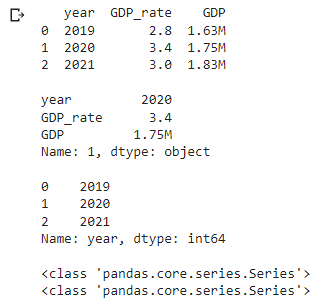# 8
import pandas as pd
s1 = pd.Series( [2019, 2020, 2021] )
s2 = pd.Series( [2.8, 3.2, 3.0] )
s3 = pd.Series( [“1.63M”, “1.75M”, “1.87M”] )
data = {“year” : s1, “GDP_rate” : s2, “GDP” : s3}
df1 = pd.DataFrame(data)
print(df1, “\n”)
names = pd.Series([“禅雅塔”, “奥丽莎”, “西格玛”, “索洁恩”, “卢西奥”])
scores = pd.Series([18030, 5069, 8958, 14560, 6800])
members = {“[姓名]”: names, “[伤害量]” : scores}
df2 = pd.DataFrame(members)
print(df2, “\n”)
df2[“[表现]”] = [“A”, “C”, “B”, “A”, “D”] # 列名以 Grade 添加列
df2.loc = [“麦克雷”, 15035, “A”] # 添加行
print(df2)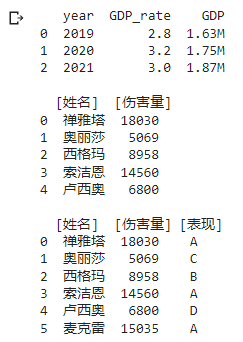我们之前提到了，numpy 也是可以塞里面的，我们来看看。

import numpy as np
import pandas as pd
data1 = np.array(
[ [1, 2, 3], [4, 5, 6] ] # 创建一个二维数组
)
df1 = pd.DataFrame(data1)
print(df1, “\n”)
data2 = pd.Series(
{“苹果”: “Apple”, “橙子”: “Orange”,
“香蕉”: “banana”, “桃子”: “peach”}
)
df2 = pd.DataFrame(data2, columns = [“英文名”])
print(df2, “\n”)
df3 = pd.DataFrame (
data = [56, 90, 31],
index = range(1, 4),
columns = [“价格”]
)
print(df3, “\n”)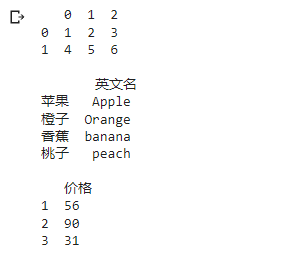### 索引与数据选择df[列名]
df.列名1
df[[列名1, 列名2, …]] # 如果有多行要引用，可以写到列表里

`df[起始位置, 结束位置]      # 从 start 开始到 end-1 行`

df.loc[行名]
df.loc[[行名1, 行名2, …]] # 如果有多行要引用，可以写到列表里

`df.loc[行名, 列名]`

`df[条件式]      # 仅引用满足条件表达式的数据`

import pandas as pd
names = [“Bob”, “Jessica”, “Mary”, “John”, “Kate”]
scores = [56, 11, 99, 83, 45]
members = {“Name”: names, “Score”: scores}
df = pd.DataFrame(data=members)
cgpa = pd.Series (
[3.2, 2.5, 4.1, 2.8, 1.9]
)
df[“Cgpa”] = cgpa
## 整体
print(df, “\n”)
print(“—— 列值引用 ——“)
print(df[[“Name”, “Cgpa”]], “\n”)
print(“—— 切片引用 ——“)
print(df[1 : 4], “\n”)
print(“—— 行引用 ——“)
print(df.loc[[2, 4]], “\n”)
print(df.loc[1, “Name”], “\n”)
print(“—— 布尔索引 ——“)
print(df[df.Score > 60]) # 筛出分数大于60的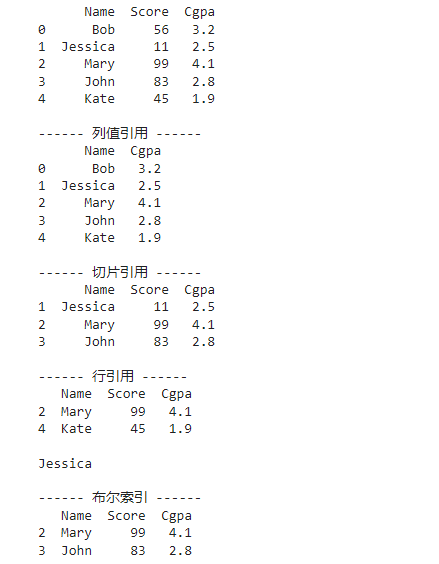loc 指的是 location，iloc 中的 i 是指的是 integer，这两者的区别如下：

• loc：根据实际设置的 index 来索引数据
• iloc：根据顺序数字来索引数据

import pandas as pd
names = [“Bob”, “Jessica”, “Mary”, “John”, “Kate”]
scores = [56, 11, 99, 83, 45]
members = {“Name”: names, “Score”: scores}
df = pd.DataFrame(data = members, index = range(1, 6))
print(df, “\n”)
## 与 df[“Name”] 相同
print(df.loc, “\n”)
print(df.iloc, “\n”)
print(df.Name)

运行结果：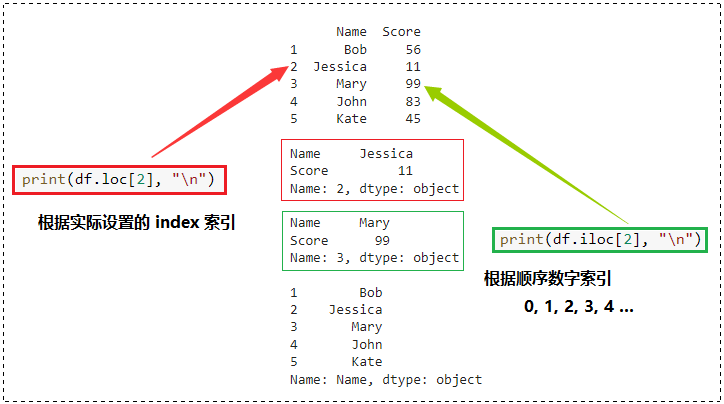### 获取对数据进行排序的新对象

#P13
import pandas as pd
names = [“Bob”, “Jessica”, “Mary”, “John”, “Kate”]
scores = [56, 11, 99, 83, 45]
members = {“Name”: names, “Score”: scores}
df = pd.DataFrame(members)
df[“Grade”] = [“C”, “F”, “A”, “B”, “D”]
df.loc = [“Hanna”, 90, “A”]
print(df, “\n”)
## 创建按 “Score” 列降序排序的对象
df1 = df.sort_values(by = “Score”, ascending = False)
print(df1, “\n”)
## 创建初始化 df1 对象索引的新对象
df2 = df1.reset_index(drop = True)
print(df2)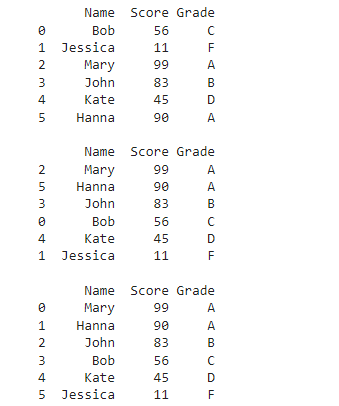### DataFrame 为对象增加新列

# p14
import pandas as pd
import numpy as np
names = [“Bob”, “Jessica”, “Mary”, “John”, “Kate”]
scores = [56, 11, 99, 83, 45]
members = {“Name”: names, “Score”: scores}
df = pd.DataFrame(members)
df.loc[2, “Grade”] = “A” # 在现有行的追加的列中储存数据
df.loc[5, “Grade”] = “F” # 追加新的行，在指定列中储存数据，剩下的数据以 Nan 形式初始化
print(df, “\n”)

运行结果：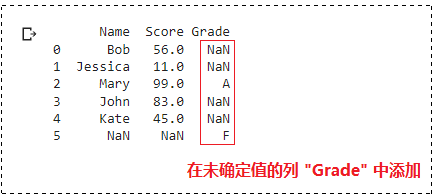import pandas as pd
import numpy as np
a = np.array([1, 2, np.nan, 4, 5])
print(a, “\n”)
print(a.sum(), a.min(), a.max())
print(np.nansum(a), np.nanmin(a), np.nanmax(a)) # 对非 nan 值计算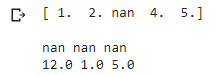### 读取外部文件数据（CSV文件）pandas 可以读取和写入各种外部数据，包括 CSV 文件、Excel 文件和文本文件。

`read_csv("文件名")`

CSV 文件以逗号（，）分隔数据，并由表示记录的行和表示字段的列组成。

import pandas as pd
print(pf, “\n”)
print(pf1, “\n”)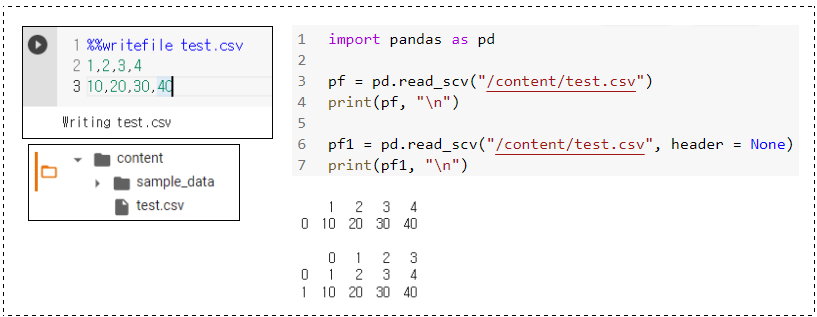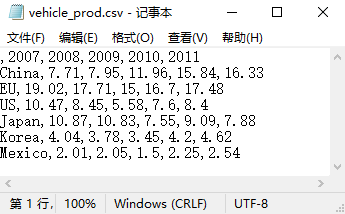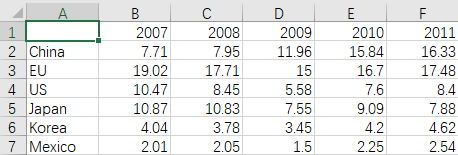（若要将第一行用作索引，省略第1行第1列的名称）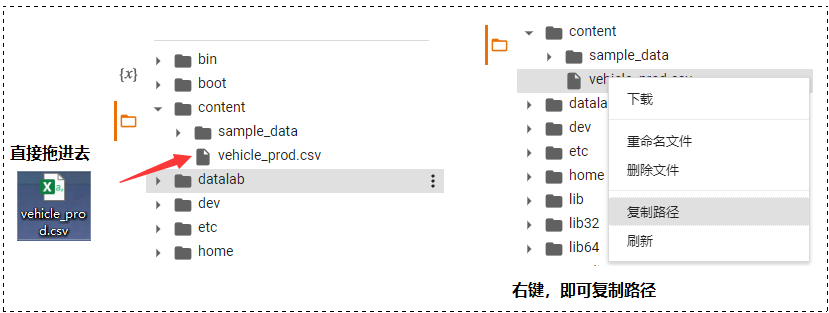import pandas as pd
import matplotlib.pyplot as plt
print(pf_f1, “\n”)
## 将第0列设置为index
pf_f2 = pd.read_csv(“/content/vehicle_prod.csv”, index_col = 0)
print(pf_f2)
pf_f2[“2007”].plot(kind=’bar’) # 将列”2007″的数据可视化
plt.show()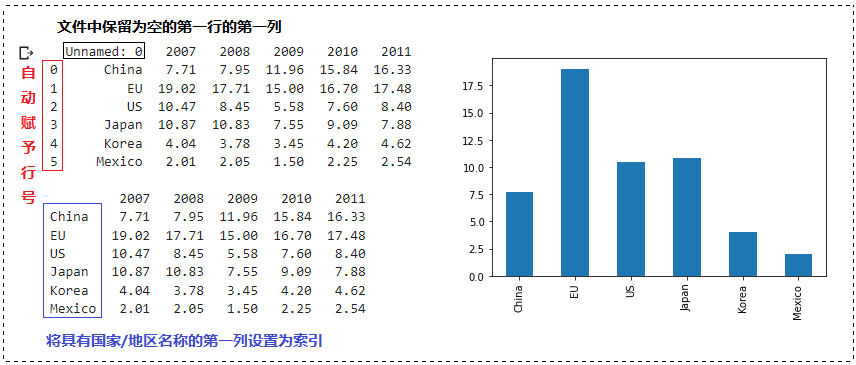import pandas as pd
import matplotlib.pyplot as plt
pf_f = pd.read_csv(“/content/vehicle_prod.csv”, index_col = 0)
plt.plot(pf_f.loc[“China”])
plt.plot(pf_f.loc[“EU”])
plt.plot(pf_f.loc[“US”])
plt.plot(pf_f.loc[“Japan”])
plt.plot(pf_f.loc[“Korea”])
plt.plot(pf_f.loc[“Mexico”])
plt.legend([“China”, “EU”, “US”, “Japan”, “Korea”, “Mexico”])
plt.show()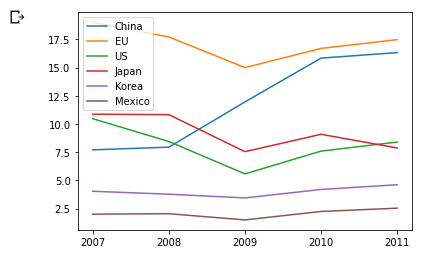import pandas as pd
import matplotlib.pyplot as plt
## 函数的参数axis=1表示列方向，axis=0表示行方向
pf_f[“Total”] = pf_f.sum(axis=1)
pf_f[“Average”] = pf_f[[“2007”, “2008”, “2009”, “2010”, “2011”]].mean(axis=1)
print(pf_f, “\n”)
## 追加列
pf_f[“Average”].plot(kind=’bar’)
plt.show()
plt.bar(pf_f.index, pf_f[“Average”])
plt.show()